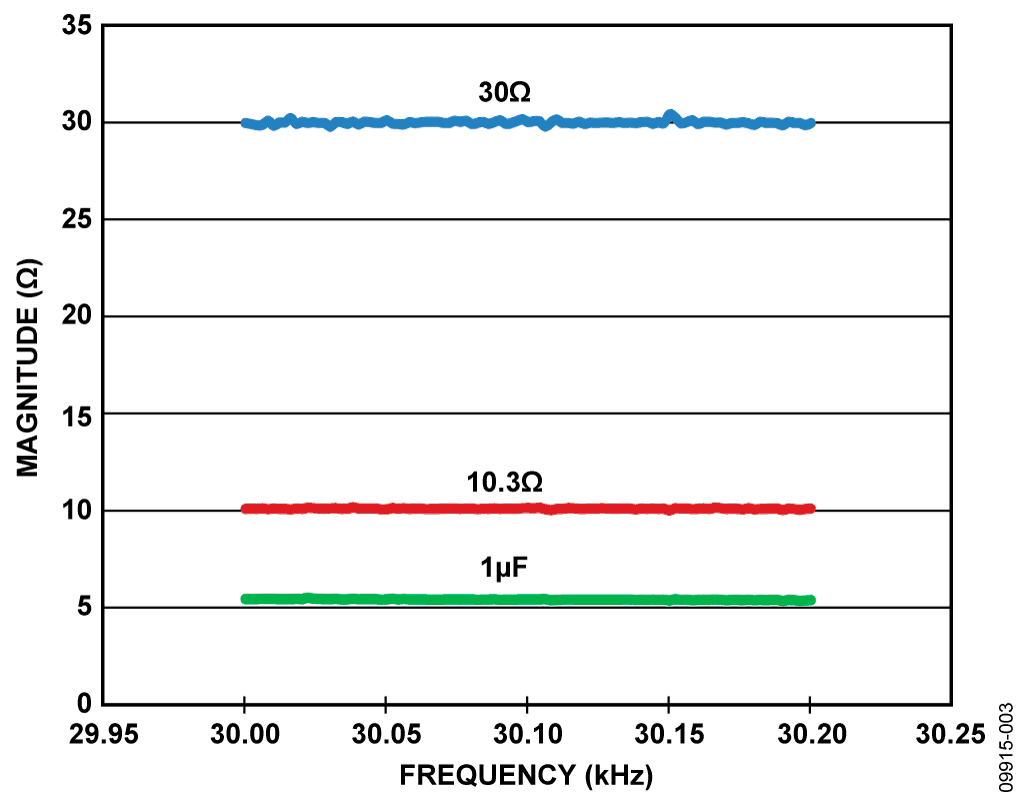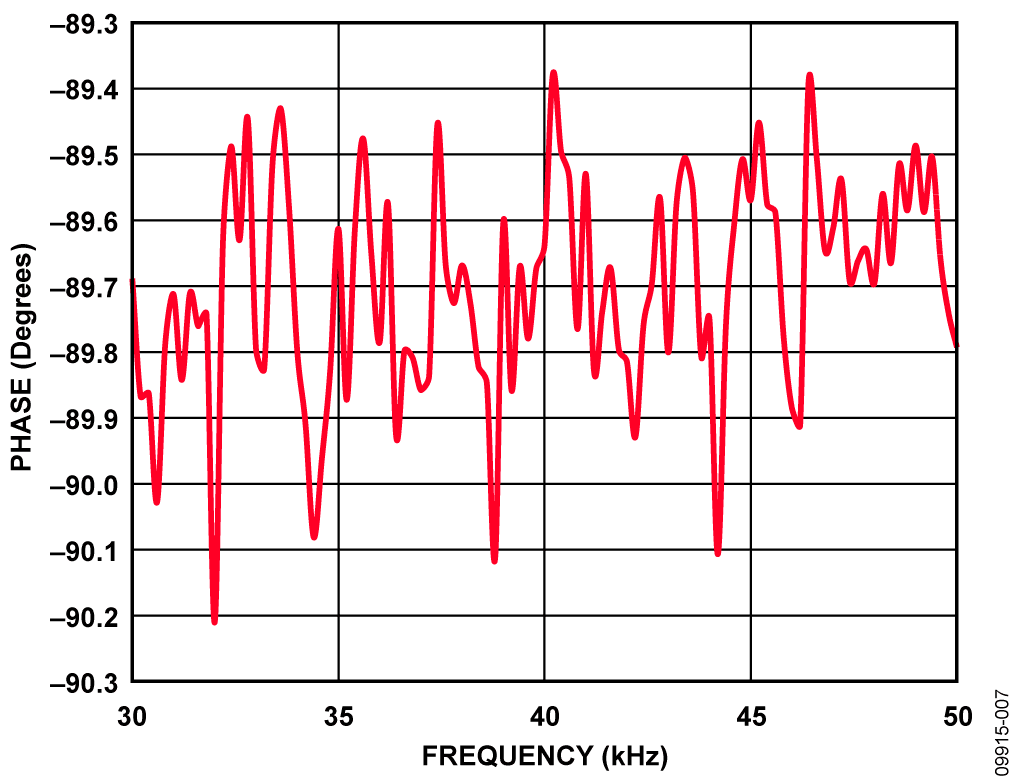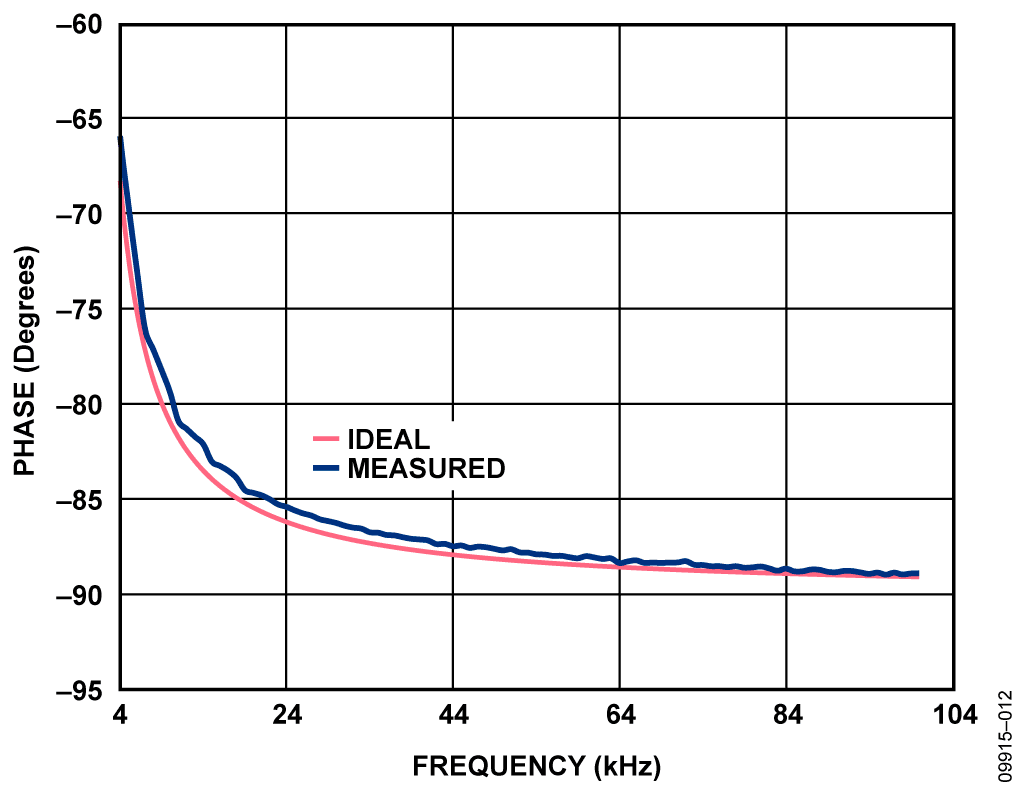Оценочные платы \$60.77## Ресурсы проектирования

### Файлы проектирования и интеграции

• Schematic
• Bill of Materials
• Gerber Files
• PADS Files
• Assembly Drawing
Загрузить файлы проектов 777 kB

### Оценочные платы

Буква "Z" в наименовании компонента указывает на соответствие требованиям RoHS. Отмеченные платы нужны для оценки данной схемы

• EVAL-AD5933EBZ (\$60.77) High Accuracy Impedance Measurements Using 12-Bit Impedance Converters

### Драйверы устройств

ПО (код на С и/или FPGA) для связи с цифровым интерфейсом компонента.

## Особенности и преимущества

• High accuracy impedance measurement
• Complex impedance measurments using internal frequency generator
• Measure up to several hundred k-ohms

## Функции и преимущества схемы

The AD5933 and AD5934 are high precision impedance converter system solutions that combine an on-chipprogrammable frequency generator with a 12-bit, 1 MSPS (AD5933) or 250 kSPS (AD5934) analog-to-digital converter (ADC). The tunable frequency generator allows an external complex impedance to be excited with a known frequency.

The circuit shown in Figure 1 yields accurate impedance measurements extending from the low ohm range to several hundred kΩ and also optimizes the overall accuracy of the AD5933/AD5934.Figure 1. Optimized Signal Chain for Impedance Measurement Accuracy (Simplified Schematic, All Connections and Decoupling Not Shown)

## Описание схемы

The AD5933 and AD5934 have four programmable output voltage ranges; each range has an output impedance associated with it. For example, the output impedance for a 1.98 V p-p output voltage is typically 200 Ω (see Table 1).

 Range Output Excitation Amplitude Output Resistance, ROUT Range 1 1.98V p-p 200 Ω typ Range 2 0.97V p-p 2.4 kΩ typ Range 3 0.383V p-p 1.0 kΩ typ Range 4 0.198V p-p 600Ω typ

This output impedance impacts the impedance measurement accuracy, particularly in the low kΩ range, and should be taken into account when calculating the gain factor. Please refer to the AD5933 or AD5934 data sheets for more details on gain factor calculation. A simple buffer in the signal chain prevents the output impedance from affecting the unknown impedance measurement. A low output impedance amplifier should be selected with sufficient bandwidth to accommodate the AD5933/AD5934 excitation frequency. An example of the low output impedance achievable is shown in Figure 2 for the AD8605/ AD8606/ AD8608 family of CMOS op amps. The output impedance for this amplifier for an AV of 1 is less than 1 Ω up to 100 kHz, which is the maximum operating range of the AD5933/AD5934.Figure 2. Output Impedance of AD8605/AD8606/AD8608

### Matching the DC Bias of Transmit Stage to Receive Stage

The four programmable output voltage ranges in the AD5933/ AD5934 have four associated bias voltages (Table 2). For example, the 1.98 V p-p excitation voltage has a bias of 1.48 V. However, the current-to-voltage (I-V) receive stage of the AD5933/AD5934 is set to a fixed bias of VDD/2 as shown in Figure 1. Thus, for a 3.3 V supply, the transmit bias voltage is 1.48 V, while the receive bias voltage is 3.3 V/2 = 1.65 V. This potential difference polarizes the impedance under test and can cause inaccuracies in the impedance measurement.

One solution is to add a simple high-pass filter with a corner frequency in the low Hz range. Removing the dc bias from the transmit stage and re-biasing the ac signal to VDD/2 keeps the dc level constant throughout the signal chain.

 Range Output Excitation Amplitude Output DC Bias Level 1 1.98V p-p 1.48V 2 0.97V p-p 0.76V 3 0.383V p-p 0.31V 4 0.198V p-p 0.173V

### Selecting an Optimized I-V Buffer for the Receive Stage

The current-to-voltage (I-V) amplifier stage of the AD5933/AD5934 can also add minor inaccuracies to the signal chain. The I-V conversion stage is sensitive to the amplifier's bias current, offset voltage, and CMRR. By selecting the proper external discrete amplifier to perform the I-V conversion, the user can choose an amplifier with lower bias current and offset voltage specifications along with excellent CMRR, making the I-V conversion more accurate. The internal amplifier can then be configured as a simple inverting gain stage.

Selection of resistor RFB still depends on the gain through the system as described in the AD5933/AD5934 data sheet.

### Optimized Signal Chain for High Accuracy Impedance Measurements

Figure 1 shows a proposed configuration for measuring low impedance sensors. The ac signal is high-pass filtered and rebiased before buffering with a very low output impedance amplifier. The I-V conversion is completed externally before the signal returns to the AD5933/AD5934 receive stage. Key specifications that determine the required buffer are very low output impedance, single-supply capability, low bias current, low offset voltage, and excellent CMRR performance. Some suggested parts are the ADA4528-1, AD8628/AD8629, AD8605, and AD8606. Depending on board layout, use a single-channel or dual-channel amplifier. Use precision 0.1% resistors for both the biasing resistors (50 kΩ) and gain resistors (20 kΩ and RFB) to reduce inaccuracies.

## Основные варианты исполнения

Other op amps can be used in the circuit, such as the ADA4528-1, AD8628, AD8629, AD8605, and the AD8608.

### Switching Options for System Applications

For this particular circuit, the ZUNKNOWN and RCAL were interchanged manually. However, in production, a low on-resistance switch should be used. The choice of the switch depends on how large the unknown impedance range is and how accurate the measurement result needs to be. The examples in this document use just one calibration resistor, and so a low on-resistance switch such as the ADG849 can be used as shown in Figure 13. Multichannel switch solutions such as the quad ADG812 can also be used. The errors caused by the switch resistance on the ZUNKNOWN are removed during calibration, but by choosing a very low RON switch, the effects can be further minimized.Figure 13. Switching Between RCAL and Unknown Z Using the ADG849 UltraLow RON SPDT Switch (Simplified Schematic, All Connections and Decoupling Not Shown)

## Оценивание характеристик и тестирование схем

The schematic in Figure 1 was developed to improve impedance measurement accuracy, and some example measurements were taken. The AD8606 dual channel amplifier buffers the signal on the transmit path and converts the receive signal from current to voltage. For the three examples shown, the gain factor is calculated for each frequency increment to remove frequency dependent errors. A complete design package including schematics, bill of materials, layout, and Gerber files is available for this solution at http://www.analog.com/CN0217-DesignSupport. The software used is the same software that is available with evaluation boards and is accessible from the AD5933 and AD5934 product pages.

### Example 1: Low Impedance Range

 Parameter Value V p-p 1.98V (Range 1) Number of Settling Time Cycles 15 MCLK 16 MHz RCAL 20.1Ω RFB 20.0Ω Excitation Frequency Range 30 kHz to 30.2 kHz Unknown Impedances R1 = 10.3Ω, R2 = 30.0Ω, C3 = 1 µF (ZC = 5.3Ω< at 30 kHz)

The results of the low impedance measurements are shown in Figure 3, Figure 4, and Figure 5. Figure 5 is for the 10.3 Ω measurement and is shown on an expanded vertical scale.

The accuracy achieved is very much dependent on how large the unknown impedance range is relative to the calibration resistor, RCAL. Therefore, in this example, the unknown impedance of 10.3 Ω measured 10.13 Ω, an approximate 2% error. Choosing an RCAL closer to the unknown impedance achieves a more accurate measurement; that is, the smaller the unknown impedance range is centered around RCAL is, the more accurate the measurement. Consequently, for large unknown impedance ranges, it is possible to switch in various RCAL resistors to break up the unknown impedance range using external switches. The RON error of the switch is removed by calibration during the RCAL gain factor calculation. Using a switch to select various RFB values can optimize the dynamic range of the signal seen by the ADC.

Also note that to achieve a wider range of measurements a 200 mV p-p range was used. If the unknown Z is a small range, a larger output voltage range can be used to optimize the ADC dynamic range.Figure 3. Measured Low Impedance Magnitude ResultsFigure 4. Measured Low Impedance Phase ResultsFigure 5. Measured 10.3 Ω Magnitude Results (Expanded Scale)

### Example 2: kΩ Impedance Range

Using an RCAL of 99.85 kΩ, a wide range of unknown impedances were measured according to the setup conditions listed in Table 2. Figure 6 to Figure 10 document accuracy results. To improve the overall accuracy, select an RCAL value closer to the unknown impedance. For example, in Figure 9, an RCAL closer to the ZC value of 217.5 kΩ is required. If the unknown impedance range is large, use more than one RCAL resistor.

 Parameter Value V p-p 0.198V (Range 4) Number of Settling Time Cycles 15 MCLK 16 MHz RCAL 99.85 kΩ RFB 100 kΩ Excitation Frequency Range 30 kHz to 50 kHz Unknown Impedances R0 = 99.85 kΩ R1 = 29.88 kΩ R2 = 14.95 kΩ R3 = 8.21 kΩ R4 = 217.25 kΩ C5 = 150 pF (ZC = 26.5 kΩ at 40 kHz) C6 = 47pF (ZC = 84.6 kΩ at 40 kHz)Figure 6. Magnitude Result for ZC = 47 pF, RCAL = 99.85 kΩFigure 7. Phase Result for ZC = 47 pF, RCAL = 99.85 kΩFigure 8. ZC = 8.21 kΩ, RCAL = 99.85 kΩFigure 9. ZC = 217.25 kΩ, RCAL = 99.85 kΩFigure 10. Magnitude Results for Example 2: R1, R2, R3, C5, C6

### Example 3: Parallel R-C (R||C) Measurement

An R||C type measurement was also made using the configuration, using an RCAL of 1 kΩ, an R of 10 kΩ, and a C of 10 nF, measured across a frequency range of 4 kHz to 100 kHz. The magnitude and phase results versus ideal are plotted in Figure 11 and Figure 12.

 Parameter Value V p-p 0.383V (Range 3) Number of Settling Time Cycles 15 MCLK 16 MHz RCAL 1 kΩ RFB 1 kΩ Excitation Frequency Range 4 kHz to 100 kHz Unknown Impedance R||C R = 10 kΩ C = 10 nFFigure 11. Magnitude Results for ZC = 10 kΩ||10 nF, RCAL = 1 kΩFigure 12. Phase Results for ZC = 10 kΩ||10 nF, RCAL = 1 kΩ

### Setup and Test

The evaluation software is that used on the EVAL-AD5933EBZ application board. Please refer to the technical note available on the CD provided for details on the board setup. Note that there are alterations to the schematic. Link connections on the EVAL-AD5933EBZ board are listed below in Table 4. Also note that the location for RFB is located at R3 on the evaluation board, and the location for ZUNKNOWN is C4.

Complete setup and operation for the hardware and software for the evaluation board can be found in User Guide UG-364.

 Link Number Default Position LK1 Open LK2 Open LK3 Insert LK4 Open LK5 Insert LK6 A

## Образцы

### Образцы

 Продукт Описание Доступный продукт Модели для образца AD8606 Precision, Low Noise, RRIO, CMOS Op Amp (Dual) AD8606ACBZ-REEL7 AD8606ACBZ-REEL7 AD8606ARMZ-R7 AD8606ARMZ-R7 AD8606ARZ AD8606ARZ AD5934 12-разрядный анализатор цепей/преобразователь импеданса с быстродействием 250 kSPS AD5934YRSZ AD5934YRSZ AD5933 12-разрядный анализатор цепей/преобразователь импеданса с быстродействием 1 MSPS AD5933WYRSZ-REEL7 AD5933WYRSZ-REEL7 AD5933YRSZ AD5933YRSZ
Функционирование раздела Покупка возможно только в полной версии сайта
Оценочные платы Цена указана за одну единицу.
Через сайт Analog.com можно приобрести не более двух оценочных плат. Чтобы заказать более двух оценочных плат, пожалуйста, совершайте покупку через наших дистрибьюторов.
Цены указаны за одну штуку, в долларах США, на условиях ФОБ. Являются рекомендованными розничными ценами в США, приведены только для примерного расчета и могут меняться. Международные цены могут отличаться на величину местных пошлин, налогов, сборов и курсов валют.